How Cheenta works to ensure student success?
Explore the Back-Story

# Test of Mathematics Solution Subjective 90 - Graphing InequalityThis is a Test of Mathematics Solution Subjective 90 (from ISI Entrance). The book, Test of Mathematics at 10+2 Level is Published by East West Press. This problem book is indispensable for the preparation of I.S.I. B.Stat and B.Math Entrance.

Also visit: I.S.I. & C.M.I. Entrance Course of Cheenta

## Problem:

:
Draw the region of points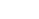in the plane, which satisfy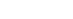.

## Solution:region will bounded by lines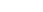,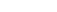,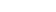&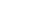. Why is that?

First note that ( |x| \le 1 ) implies:Similarly, if we demand ( |y| \le 1 ) (the double shaded zone).Now if we want ( |y| \le |x| ) . This can be achieved by

•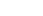when x and y are both positive (in the first quadrant); that is the region below the line x = y
•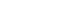when x is negative and y positive (in the second quadrant); hence the region below the line y = -x
•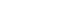when (x, y) is in the third quadrant.
•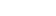when (x, y) is in fourth quadrant.

Therefore the final region is the following shaded region:This is a Test of Mathematics Solution Subjective 90 (from ISI Entrance). The book, Test of Mathematics at 10+2 Level is Published by East West Press. This problem book is indispensable for the preparation of I.S.I. B.Stat and B.Math Entrance.

Also visit: I.S.I. & C.M.I. Entrance Course of Cheenta

## Problem:

:
Draw the region of pointsin the plane, which satisfy.

## Solution:region will bounded by lines,,&. Why is that?

First note that ( |x| \le 1 ) implies:Similarly, if we demand ( |y| \le 1 ) (the double shaded zone).Now if we want ( |y| \le |x| ) . This can be achieved by

•when x and y are both positive (in the first quadrant); that is the region below the line x = y
•when x is negative and y positive (in the second quadrant); hence the region below the line y = -x
•when (x, y) is in the third quadrant.
•when (x, y) is in fourth quadrant.

Therefore the final region is the following shaded region:### Knowledge Partner